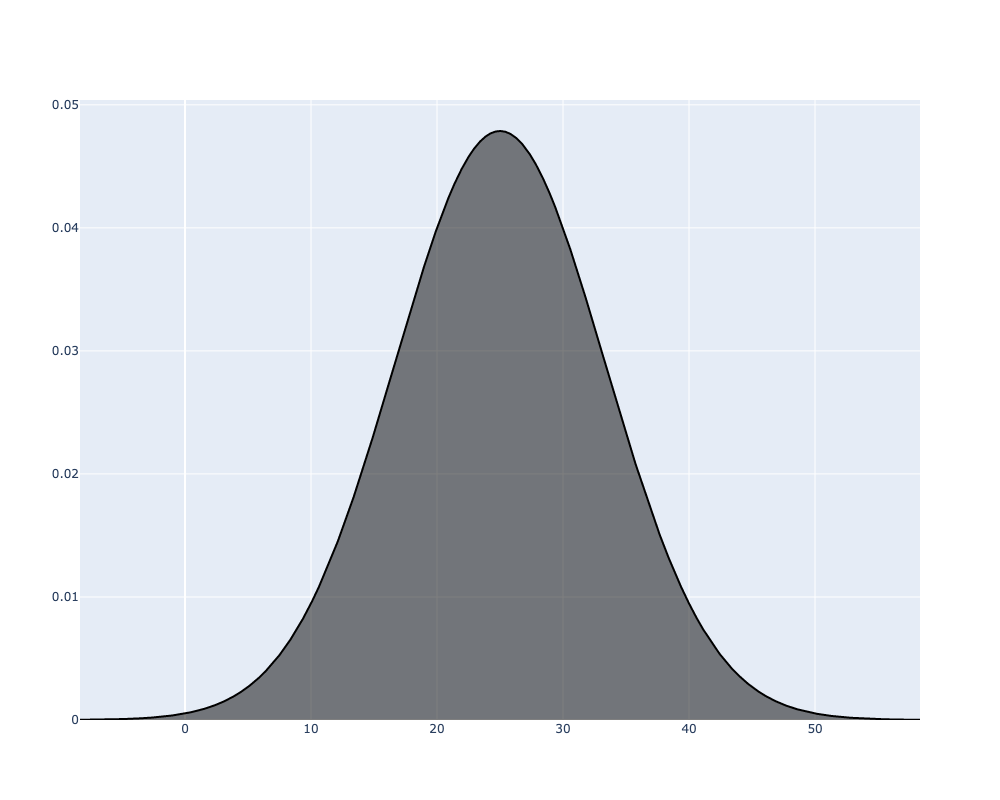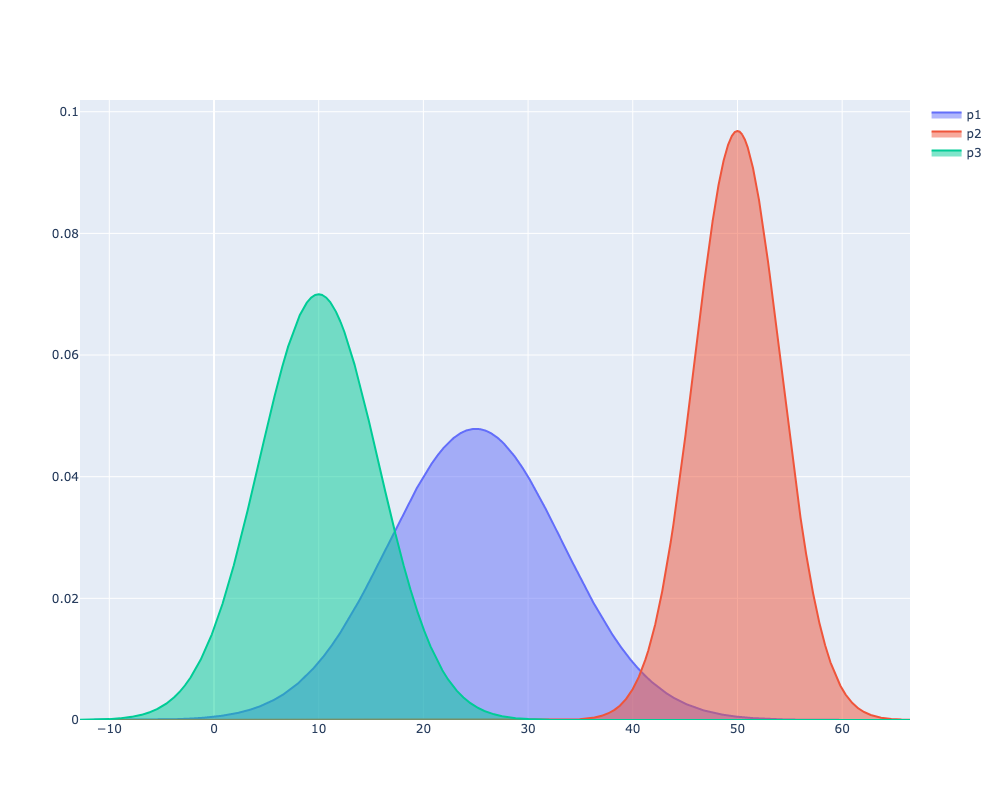# Plotly: Visualising a normal distribution given average and standard deviation

I’ve been playing around with Microsoft’s TrueSkill algorithm, which attempts to quantify the skill of a player using the Bayesian inference algorithm. A rating in this system is a Gaussian distribution that starts with an average of 25 and a confidence of 8.333. I wanted to visualise various ratings using Plotly and that’s what we’ll be doing in this blog post.

To save you from having to install TrueSkill, we’re going to create a named tuple to simulate a TrueSkill `Rating` object:

``````from collections import namedtuple
Rating = namedtuple('Rating', ['mu', 'sigma'])

base = Rating(25, 25/3)``````

If we print that object, we’ll see the following output:

Output
``Rating(mu=25, sigma=8.333333333333334)``

To create a visualisation of this distribution, we’ll need to install the following libraries:

``pip install plotly numpy scipy kaleido==0.2.1``

Next, import the following libraries:

``````import numpy as np
from scipy.stats import norm
import plotly.graph_objects as go``````

Next, we’re going to create some values for the x-axis. We’ll use numpy’s `arange` function to create a series of values starting from -4 standard deviations to +4 standard deviations:

``x = np.arange(base.mu-4*base.sigma, base.mu+4*base.sigma, 0.001)``
Output
``````array([-8.33333333, -8.33233333, -8.33133333, ..., 58.33066667,
58.33166667, 58.33266667])``````

Next, we’re going to run scipy’s probability density function over the x values for our mu and sigma values. In other words, we’re going to compute the likelihood of each of those x values for our normal distribution:

``y = norm.pdf(x, base.mu, base.sigma)``
Output
``````array([1.60596271e-05, 1.60673374e-05, 1.60750513e-05, ...,
1.60801958e-05, 1.60724796e-05, 1.60647669e-05]``````

Now that we’ve got x and y values, we can create a visualisation by running the following code:

``````fig = go.Figure()
fig.write_image("fig1.png", width=1000, height=800)``````

If we run that code, the following image will be generated:Figure 1. Normal distribution with mu=25, sigma=8.33

We can then wrap that all together into a function that can take in multiple ratings:

``````def visualise_distribution(**kwargs):
fig = go.Figure()
min_x = min([r.mu-4*r.sigma for r in kwargs.values()])
max_x = max([r.mu+4*r.sigma for r in kwargs.values()])
x = np.arange(min_x, max_x, 0.001)
for key, value in kwargs.items():
y = norm.pdf(x, value.mu, value.sigma)
``visualise_distribution(p1=Rating(25, 8.333), p2=Rating(50, 4.12), p3=Rating(10, 5.7))``We can see that the curve for `p2` is much narrower than the other two, which is because we used a small sigma value. `p1` is the default score and as a result we have the most uncertainty in that rating. `p3` hasn’t performed well and there’s reasonably certainty around their low score.